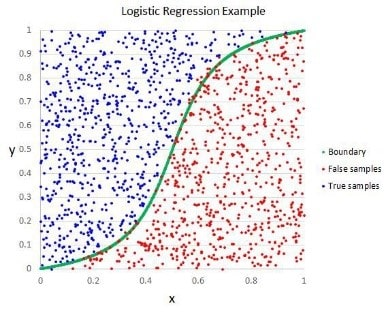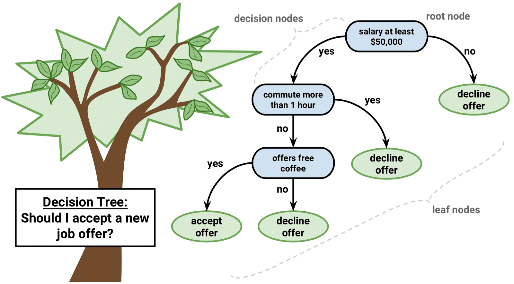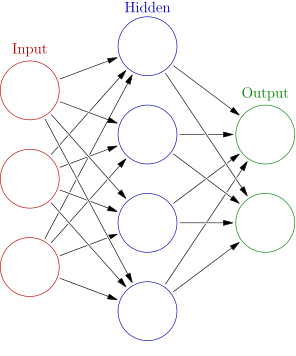## Machine Learning: Choosing a Machine Learning Model

As more companies look to leverage their data using the predictive capabilities of machine learning, they find that there is no one size fits all approach to this exciting technology. The machine learning algorithm you choose depends on the size, quality, and type of data as well as the project timeline and your overall goals. Choosing the proper machine learning algorithm lends context to the insights gained from the resulting predictions.

The following are a few considerations to take into account when beginning a machine learning project:

Accuracy: Is the goal of your project to determine the most accurate result or will an approximation satisfy your project needs? Approximating outputs can reduce processing time and keep performance high for large datasets.

Training Time: The amount of training time needed varies between machine learning algorithms and can also vary by the desired level of accuracy.

Linearity: Many machine learning algorithms assume the input data is linear meaning these models will assume data classifications can be separated along a straight line or that the data follows a linear trend. Linear models can be trained quickly, but the assumption that the underlying data is linear can lead to reduced accuracy if the dataset actually follows a non-linear trend.

Features: Features are the attributes of your dataset (think of them as the column headings in a spreadsheet), and a large set of features can also hinder the performance of a machine learning model.

## Types of Machine Learning Algorithms

After consideration of your data properties and the project requirements and goals, you can begin to map those requirements to the best machine learning model. A description of a few different types of machine learning algorithms can be found below:

### Linear RegressionLinear regression is a popular and well-studied model that can be trained quickly making this a good starting point for a machine learning project. This algorithm can provide accurate predictions, but it assumes data linearity (so this may not be the best model for non-linear datasets). If possible and appropriate for the project, outliers should be removed from the dataset to improve speed and performance. Linear regression models will provide the resulting outputs on a continuous scale.

### Logistic Regression

Similar to a linear regression, a logistic regression can typically be trained quickly and provide highly accurate results especially when unrelated features or data outliers are removed. Unlike linear regression, use of a logistic regression model results in a discrete classification (i.e. true or false, spam email or not spam email) of values using a non-linear function compared to providing results on a continuous scale.

### Decision TreesThe decision tree model features decision nodes representing individual variables. These decision nodes split either into another decision node and a leaf node or two leaf nodes. The leaf nodes represent the outcome of the decision. These outputs are used to make future predictions. Decision tree models require little data preparation and can accurately predict a wide range of outcomes.

### Bagging and Random Forest

Bagging is a technique that creates an average based on multiple models (usually decision trees) from multiple samples of the training dataset. New data is run through all models, and the predictions are averaged with the aim of improving output accuracy. While Bagging focuses on optimal outcomes, Random Forest uses randomness to generate suboptimal splits from a decision node. Combining the predictions from bagging and random forest models can provide a better estimate of the true output value increasing project accuracy.

### Artificial Neural NetworkArtificial Neural Networks are modeled after learning in the human brain. This technique can be geared toward complex pattern recognition. An Artificial Neural Network creates a connection of nodes which map an input to an output through one to multiple hidden layers. This creates complex feature detectors in a nonlinear environment identifying hidden patterns that would be impossible to predict through human analysis.

## Final Thoughts

No one machine learning algorithm will be the answer to every problem. Your project goals and the available data impact the best approach for machine learning. A consultant can help guide you through the goal setting process to better determine the machine learning model to meet that goal. They can determine potential issues like data quality and present solutions and implementation to overcome challenges. UDig consultants help leverage historical business data to develop a model that returns the insights that lead to actionable results.

## DIG IN > Data